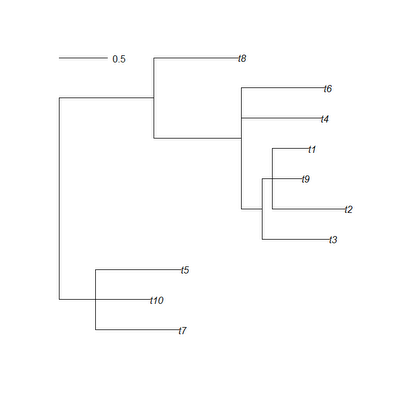## Saturday, March 17, 2012

### Trick to get the mean height of internal nodes

Here's a quick trick I came up with while working on something else today that gives the mean height of internal nodes (above the root) on a phylogenetic tree.

First, some quick background. The phytools function nodeHeights computes the height above the root for all the nodes and tips of the tree, and it returns them in a matrix of dimensions tree\$edge [the matrix containing the parent (left column) and daughter (right column) node numbers of your tree]. So, for instance:

> require(phytools)
> tree<-rtree(10)> H<-nodeHeights(tree)
> H
[,1]      [,2]
[1,] 0.0000000 0.3767669
[2,] 0.3767669 1.1140877
[3,] 1.1140877 1.9673950
[4,] 1.1140877 1.6751804
[5,] 0.3767669 1.2550270
[6,] 0.0000000 0.9705378
[7,] 0.9705378 1.8688737
[8,] 1.8688737 2.0820751
[9,] 2.0820751 2.7701772
[10,] 2.0820751 2.1866002
[11,] 2.1866002 2.9329200
[12,] 2.1866002 2.3545078
[13,] 2.3545078 2.6565813
[14,] 2.3545078 2.7237393
[15,] 1.8688737 1.9883786
[16,] 1.9883786 2.8042467
[17,] 1.9883786 2.8374805
[18,] 0.9705378 1.8377918

It's clear from a casual inspection of the matrix that each parent node height (in the right column) is represented twice and only twice. Thus, if we exclude the root node (zero height), we can just take the mean of H[,1].

> hbar1<-mean(sort(H[,1])[3:nrow(H)])
> hbar1
 1.617728

The only problem with this is that it will not work for trees in which some nodes have more than two descendants (i.e., multifurcating trees). Instead, we can do the following:

> hbar2<-mean(H[sapply(length(tree\$tip)+2:tree\$Nnode,match, tree\$edge[,1]),1])
> hbar2
 1.617728

This works by matching all internal node numbers, excluding the root, to the first column of tree\$edge, and then pulling only those elements out of H[,1]. Importantly, the base function match identifies the index of only the first instance of an item in a vector. (The function which can be used to pull the indices of multiple matching items from a vector.)

To see how this matters, let's collapse some of the nodes of our tree into multifurcations.

> tree\$edge.length[c(2,12,15)]<-0
> tree<-di2multi(tree)> hbar1<-mean(sort(H[,1])[3:nrow(H)])
> hbar1
 1.492458
> hbar2<-mean(H[sapply(length(tree\$tip)+2:tree\$Nnode,match, tree\$edge[,1]),1])
> hbar2
 1.496971

(OK, so it is a very small effect in this case, but it could be significant. That's what I get for not running my example all the way through before posting it.)

1.Hi Liam,

just a beginner's question. Why the values of node heights obtained by your function nodeHeights() do not has coincidence with the values estimated using ape's branching.times()? (my tree is ultrametric).

thanks!

2.ooooups sorry, I've just found the answer and embarrassed a bit myself. The values of the nodeHeights() are from root to tips, while in branching.times() are from tips to root...

3.Yup, that's exactly right. - Liam

Note: due to the very large amount of spam, all comments are now automatically submitted for moderation.